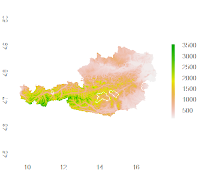## 20 Jun 2013

### Spatial Overlays with R - Retrieving Polygon Attributes for a Set of Points

A short tutorial for spatial overlays using R-GIS..

```library(sp)
library(dismo)

# spatial data (political districts of Austria)

# view

pts <- geocode(c("Aldrans, Grubenweg", "Wien, Stephansdom", "Salzburg, Mozartplatz"))

# make pts spatial
coords <- SpatialPoints(pts[, c("longitude", "latitude")])
spdf_pts <- SpatialPointsDataFrame(coords, pts)

# assign CRS/projection (which is WGS 1984)
crs <- CRS(" +proj=longlat +datum=WGS84 +ellps=WGS84 +towgs84=0,0,0")
proj4string(spdf_pts) <- crs

# check data
str(spdf_pts)

# plot pts on top
plot(spdf_pts, cex = 2, col = 2, add = T)

# do an intersection (points in polygon)
# yielding the polygon's attribute data
```

## 18 Jun 2013

### R GIS: Terrain Analysis for Polygons as Simple as it Gets!```library(rgdal)
library(raster)

alt <- getData('alt', country = "AT")

plot(alt)

asp <- terrain(alt, opt = "aspect", unit = "degrees", df = F)
slo <- terrain(alt, opt = "slope", unit = "degrees", df = F)

> extract(slo, gadm_sub, fun = mean, na.rm = T, small = T, df = T)
ID     slope
1  1  9.959053
2  2  1.047443
3  3  7.456165
4  4  1.673786
5  5 11.946553

> extract(asp, gadm_sub, fun = mean, na.rm = T, small = T, df = T)
ID   aspect
1  1 170.8065
2  2 184.0130
3  3 190.7155
4  4 136.8953
5  5 205.2115
```

Here's a little r-script to convenientely download high quality digital elevation data, i.e. for the Alps, from HERE:

```require(XML)

dir.create("D:/GIS_DataBase/DEM/")
setwd("D:/GIS_DataBase/DEM/")

doc <- htmlParse("http://www.viewfinderpanoramas.org/dem3.html#alps")
urls <- paste0("http://www.viewfinderpanoramas.org", xpathSApply(doc,'//*/a[contains(@href,"/dem1/N4")]/@href'))
names <- gsub(".*dem1/(\\w+\\.zip)", "\\1", urls)

# unzip all files in dir and delete them afterwards
sapply(list.files(pattern = "*.zip"), unzip)
```

p.s.: Also check raster::getData which pulls SRTM data at 90m resolution for a location / region!

## 7 Jun 2013

### QGIS: Curing Small Aesthetical FlawProcrastination ahead! ..When starting QGIS, does the popping up of the cmd prompt window also annoy you like me? If you want to solve this, put the below vbs script in your PATH/bin folder (or anywhere else, if you wish).

Check the path to qgis.bat in the script and change it if yours is different. Then, go to the QGIS-Desktop shortcut and in the options dialogue point to the vbs script as target. Your done - no more popping cmd windows when starting QGIS!

```Set WshShell = CreateObject("WScript.Shell")
WshShell.Run chr(34) & "C:\OSGeo4W\bin\qgis.bat" & Chr(34), 0
Set WshShell = Nothing
```